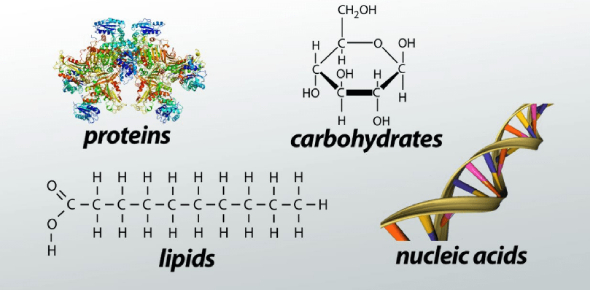25 Questions | Total Attempts: 3754SettingsBiochemistry quiz review. For me.

• 1.
Characteristic(s) of carbon that contribute to its ability to form diverse organic molecules include
• A.

Ability to bond up to four other atoms

• B.

Capacity to form single and double bonds

• C.

Ability to bond and form branched skeletons

• D.

All of the above

• 2.
If a lipid is a solid at room temperature, you would predict
• A.

All lipids are solids at room temperature

• B.

The lipid is saturated

• C.

The lipid is unsaturated

• D.

There is not enough information

• 3.
Which, if any, of the following choices does not properly pair an organic compound with one of its building blocks?
• A.

Nucleic acid -- nucleotide

• B.

Polysaccharide -- monosaccharide

• C.

Fat -- peptide

• D.

All are paired correctly

• 4.
A scientist is studying the metabolism of proteins in yeast ants to follow the formation of proteins from its earliest point. In her experiment, she will feed the yeast radioactive nutrients and follow the fate of the radioactivity in the cells. Which of the following atoms will allow her to exclusively follow proteins in the cell?
• A.

• B.

• C.

• D.

• 5.
Fat accumulates in our bodies when we overeat. This is because ________.
• A.

Lipids are found only in plants

• B.

Lipids are a form of long-term energy storage

• C.

Lipids are important for cell membranes

• D.

Lipids have enzymatic activity

• 6.
Carbohydrates ____________.
• A.

Have the general chemical formula CH2O

• B.

Function as an energy source

• C.

Can exist as monomers (monosaccharides) and polymers (polysaccharides)

• D.

All of the above

• 7.
Liquid water shows __________.
• A.

Heat resistance

• B.

Polarity

• C.

Cohesion

• D.

All of the above

• 8.
Atoms share electrons unequally in a(n) ___________ bond.
• A.

Polar covalent

• B.

Hydrogen

• C.

Ionic

• D.

Non polar covalent

• 9.
How are macromolecules synthesized?
• A.

Hydrolysis of polymers

• B.

Hydrolysis of monomers

• C.

Dehydration using monomers

• D.

Dehydration using polymers

• 10.
The molecule H2SO4 has a solution that would be _________.
• A.

Acidic, as there are more OH- than H+ ions

• B.

Acidic, as there are more H+ than OH- ions

• C.

Basic, as there are more OH- than H+ ions

• D.

Basic, as there are more H+ than OH- ions

• 11.
The tendency of water molecules to stick together _________.
• A.

Acts to moderate temperature

• B.

Provides surface tension that allows insects to walk on water

• C.

Keeps waver moving through the vessels in a tree trunk

• D.

All of the above

• 12.
What type of bond is joining the two hydrogen atoms?
• A.

Hydrogen

• B.

Covalent

• C.

Hydrogen

• D.

Hydrophobic

• 13.
Isotopes are __________.
• A.

Atoms with unequal number of protons and neutrons

• B.

Atoms with equal number of protons and electrons

• C.

Atoms with same number of protons, but different number of neutrons

• D.

None of the above

• 14.
Which of these is NOT a lipid?
• A.

Cholesterol

• B.

Wax

• C.

Steroids

• D.

RNA

• 15.
Carbohydrates are mainly used in our bodies:
• A.

Structural molecules, such as hair

• B.

For membrane construction

• C.

As fuel

• D.

For building genetic material

• 16.
What is the role of DNA and RNA in cells?
• A.

Preserving and expressing genetic information

• B.

Storing energy in fat cells

• C.

Speeding up chemical reactions

• D.

Forming long fibers, like hair

• 17.
An acid is characterized as a substance that ____________.
• A.

Removes hydrogen ions from solutions

• B.

Donates hydrogen ions to solutions

• C.

Is a versatile solvent

• D.

Forms covalent bonds with other substances

• 18.
In a hydrogen bond, a covalently bound hydrogen atom weakly attracts a(n) _________ atom in a different molecule or a different region of the same molecule
• A.

Electropositive

• B.

Electronegative

• C.

Electromagnetic

• D.

Neutral

• 19.
Proteins are polymers of __________.
• A.

Nucleotides

• B.

Hydrocarbons

• C.

Glycerol

• D.

Amino acids

• 20.
The atomic mass of an element is a weighted average of all of the different ____________ of Lithium.
• A.

Ions

• B.

Isomers

• C.

Isotopes

• D.

Compounds

• 21.
A nucleotide is composed of:
• A.

Phosphate group, nitrogen containing base, and a hydrocarbon

• B.

Glycerol, nitrogen containing base, and a five carbon sugar

• C.

Phosphate group, nitrogen containing base, and a five carbon sugar

• D.

Amino acid, a nitrogen containing base, and a five carbon sugar

• 22.
• A.

Nucleotide

• B.

Fatty acid

• C.

Simple sugar

• D.

Amino acid

• 23.
• A.

Nucleotide

• B.

Fatty acid

• C.

Simple sugar

• D.

Amino acid

• 24.
• A.

Nucleotide

• B.

Fatty acid

• C.

Simple sugar

• D.

Amino acid

• 25.
• A.

Nucleotide

• B.

Fatty acid

• C.

Simple sugar

• D.

Amino acid

Related TopicsBack to top